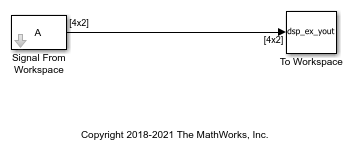# Import Two-Channel Signal From Workspace

Import a two-channel signal, `A`, from the MATLAB® workspace into the Simulink® model using the Signal From Workspace block. Use the To Workspace block to write the imported data to the MATLAB workspace. In this model, the To Workspace block writes slightly modified data to the MATLAB workspace.

The parameters in the Signal From Workspace block are configured as follows:

• Sample time set to `1`: This parameter determines, Ts , the sample period of the output.

• Samples per frame set to `4`: Number of samples, Mo , to buffer into each output frame. The output frame period is MoTs.

• Form output after final value by set to `Setting to zero`: The block generates zero-valued outputs for the duration of the simulation after generating the last frame of the signal.

Open and simulate the model.The Signal From Workspace block imports the signal from the variable A, which is a 10-by-2 matrix. When you simulate the model, the data output has a frame size of 4 and a frame period, MoTs , of 4 seconds. All outputs after the third frame (at `t = 8`) are zero because the Form output after final data value by parameter is set to `Setting to zero` in this model. The To Workspace block writes this modified output to the MATLAB workspace in the variable `dsp_ex_yout`.

This figure shows the signal in the input variable `A` and how this data is written to the output array `dsp_ex_yout`.